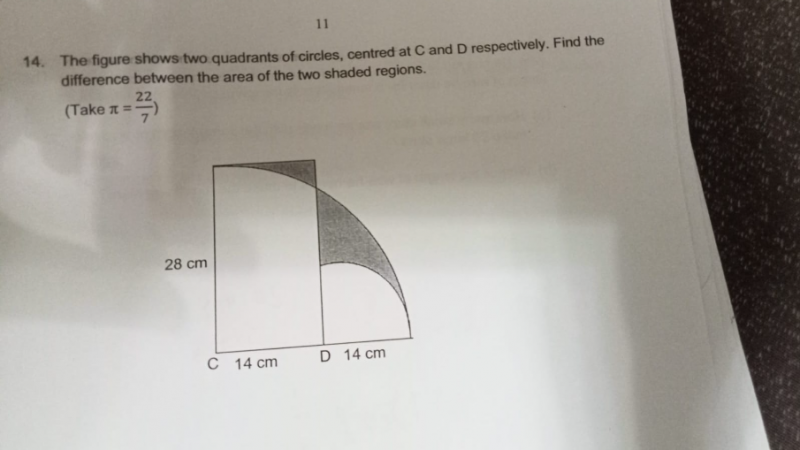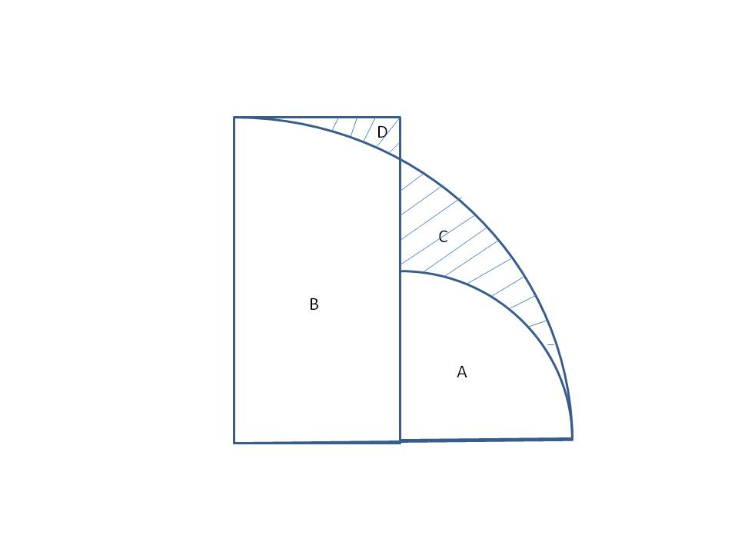# QuestionThank you.Based on the diagram above,  we can see that the question wants you to find C-D.

B + C – (B + D) = B + C – B – D = C – D

B+ C = Area of large quadrant – Area of small quadrant

B+ D = Area of rectangle

So C – D = (1/4) (22/7)(28)(28) – (1/4)(22/7)(14)(14) – (14 x 28)

= 616 – 154 – 392

= 70

Therefore, difference between the 2 shaded regions = 70cm2

0 Replies 2 Likes ✔Accepted Answer